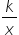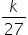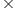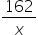Maths-
General
Easy

Question

# A certain project can be completed by 6 workers in 27 days. In order to complete the project sooner, the company plans to hire additional workers. How many workers are needed to complete the project in 18 days?Hint:

## The correct answer is: 18 days.

### Let the number of workers be represented as x and the time taken to complete the project be represented as y. Time taken to complete the project decreases if the number of workers increases so we can conclude that x is in inverse relationship with y. Let’s say the proportional relationship is given asy =……..(1)Step 1 of 2:It is given that the project can be completed by 6 workers in 27 days i.e. x = 6 and y = 27. Putting the values in equation (1)6 =k = 627  = 162Step 2 of 2:Now we are asked to determine the number of additional workers if the time taken to complete the project is 18 days. So, y = 18 and we need to find x. Putting the values in equation (1)18 =Now, put the value of k = 16218 =x =x = 9 workersNumber of additional workers = 9 - 6 = 3 workersFinal Answer: Hence, the company needs to hire 3 more additional workers to complete the project in 18 days.#### With Turito Foundation.#### Get an Expert Advice From Turito.